# Advent of Code 2020 - Day 6

Time to check for some custom declarations forms. In the first part we will check the distinct number of questions anyone on each group answered “yes”, and on the second part the questions where everyone gave a positive response.

If you want to follow along please download AdventOfCode2020.pbit and check Day 1 post for instructions.

## Part 1

The input for this problem is very similar to the passports we have seen on day 4. We have several lines that represent the answers of the persons in a group and the groups are separated by a blank line.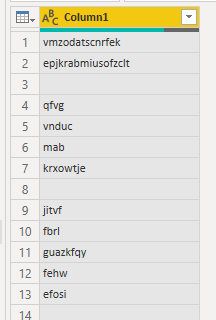Figure 1: Input

Using the same strategy as we did: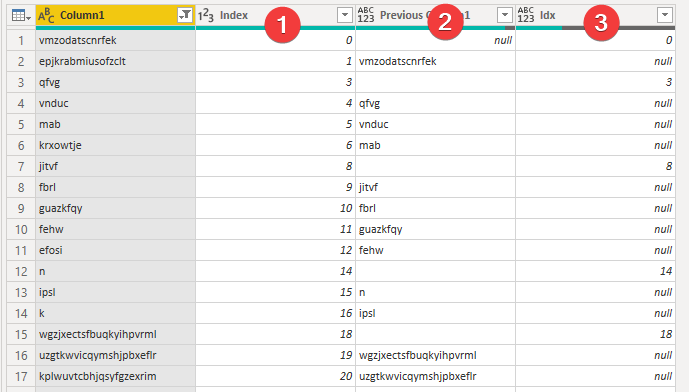Figure 2: First three steps
1. Added an index column
2. Using the index get the value of the previous row to help us know where one passport ends and the other starts
3. Add a conditional index column filled only where a blank line exists

Next we remove the empty lines on [Column1] and use the Table.FillDown on [Idx] to be ready to group by.

Now for the trick, because using the default group by button we can’t select any option to concatenate text.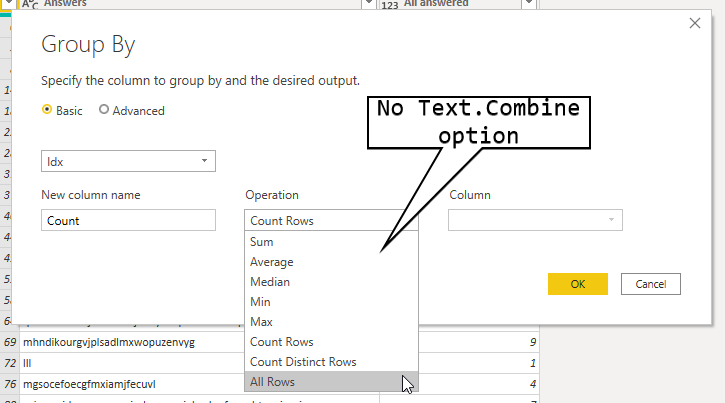Figure 3: No Text.Combine

Choose one of the options, like SUM and then in the formula bar replace the generated text with:

Text.Combine([Column1], "")


If you can’t see the formula bar go to view and select the option.

In the end we should have something like this: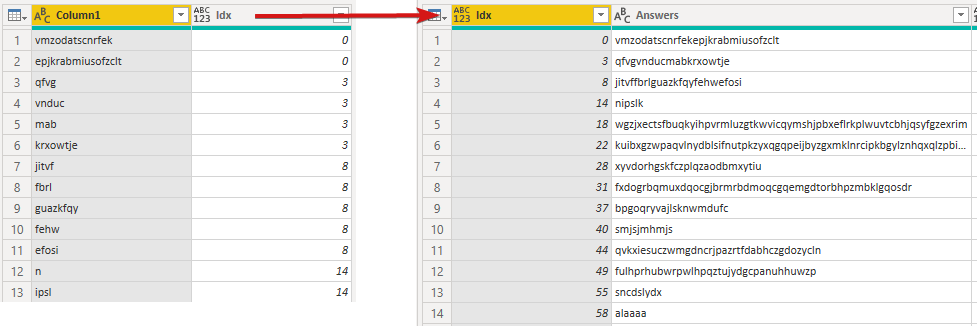Figure 4: Grouping by

All that’s left to solve this problem is to add a column with the following formula:

List.Count(List.Distinct(Text.ToList([Answers]))


Text.ToList converts the string to a list of characters, then the List.Distinct removes the duplicates and finally List.Count gives us the number we want.

All that’s left is for the DAX measure to sum the [Distinct answers] column.

## Part 2

Instead of counting the number of distinct answers we now need to count the questions where everyone answered yes. This is more tricky and I will try my best to explain what’s going on where.

The important part here is the usage of the List.Accumulate. Go and check the documentation to help you understand better what’s going on here.

We need to add this step on the Group By step we created on the previous step. If you don’t do it like this we lose the ability to intersect the answers from the elements of each group.

It should look like this: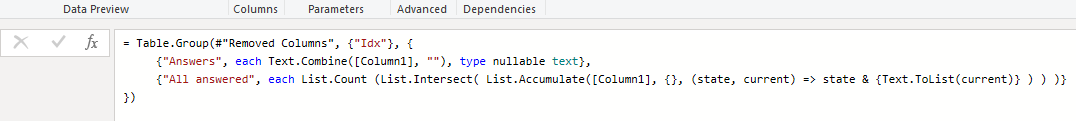Figure 5: Adding the List.Accumulate

This new column will be the length of the intersections of repeated answers in each group.

Breaking down the formula in pseudo-power-query using one of the groups given by example:

C = {[Column1="ab"], [Column1="ac"]}

List.Count(
List.Intersect(
List.Accumulate(C, {},
(state, current) => state & {Text.ToList(current)})
)
)

=>

List.Count(
List.Intersect(
List.Accumulate({"ac"}, {},
(state, current) => {} & {Text.ToList("ab")})
)
)

=>

List.Count(
List.Intersect(
List.Accumulate({}, {{"a","b"}},
(state, current) => {{"a","b"}} & {Text.ToList("ac")})
)
)

=>

List.Count(
List.Intersect({{"a","b"}, {"a", "c"}})
)

=>

List.Count({"a"})

=>

1


Once again the only thing left is to sum this new column using DAX to have the answer to the puzzle.

## Conclusion

After we have saw the equivalent of the MAP function in functional languages in Power Query, with the use of List.Transform on Day 5, now was the time to show the FOLD equivalent in the form of List.Accumulate. This allowed us to achieve an elegant solution for a tricky problem.

Have fun!!

#### Share!

comments powered by Disqus